# ACT Math Practice Test 9

Difficulty Level – 5: Very Difficult

Directions: Solve each problem and then click on the correct answer. You are permitted to use a calculator on this test.

Congratulations - you have completed .

You scored %%SCORE%% out of %%TOTAL%%.

Your performance has been rated as %%RATING%%

 Question 1
If a = 3c − 4 and b = 2(3 − c) what is 3b expressed in terms of a?

 A 10 − 2a B 5 − 2a C 10 − a D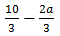E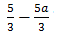Question 1 Explanation:
The correct answer is (A). Here we are given a system of equations and asked to solve in terms of one of the given variables. It is important to note that we are looking for 3b and not solving for b alone. We will begin by solving for c in terms of a using the first equation. We will then substitute this value into the second equation so as to eliminate the c variable and be left with only a’s and b’s. We will then solve for 3b: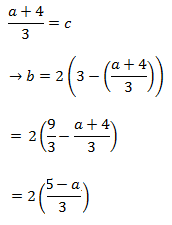Here we will multiply both sides by 3 so we have our target value of 3b on one side, and we will then simplify the expression on the other side:
3b = 2(5 − a)
= 10 − 2a
 Question 2
Let the function ƒ(h, k) be defined as ƒ(h, k) = (hk)² + hk. For every x and y, what does the function ƒ((x² + 2y²),(x² − y²)) evaluate to?

 A x4 + x²y² + 7y4 B x4 + 2x²y² + 9y4 C 2x4 + 2x²y² − 2y4 D x4 − 2x²y² − 7y4 E 2x4 − xy + 9y4
Question 2 Explanation:
The correct answer is (A). In this case we will use the definition provided to substitute values and evaluate the function:
Set h = (x²+ 2y² ) and k = (x² − y² ) and make the appropriate substitutions. Square the difference between h and k to find 9y4 and to this add the FOILED h * k − (x4 + x²y² – 2y4)) to find x4 + x²2y² + 7y4.
 Question 3
Let x > 1. What is the value of: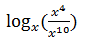A −6 B −4 C ½ D 4 E 6
Question 3 Explanation:
The correct answer is (A). This question is asking x to what power equals the value of (x4)/(x10). Evaluate (x4)/(x10) to get 1/x6 or x−6. So x must equal −6.
 Question 4
If (a² − b²)² = 25 and a²b² = 6, what is the value of a4 + b4?

 A −22 B −12 C 3 D 25 E 37
Question 4 Explanation:
The correct answer is (E). Begin by foiling the first expression:
a4 − 2a²b² + b4 = 25

We know that a²b² = 6 so make that substitution as well:
a4 −2(6) + b4 = 25
a4 + b4 = 37
 Question 5
A class contains 2 boys for every 1 girl. 75% of the boys have taken Algebra 2, whereas 50% of the girls have taken Algebra 2. What is the ratio of boys who have taken Algebra 2 to girls who have taken Algebra 2?

 A 1:1 B 1:2 C 2:1 D 3:2 E 3:1
Question 5 Explanation:
The correct answer is (E). Because we are provided only the ratio of boys to girls, we can provide our own number of boys and girls so long as it satisfies the provided ratio. We will assume there are 10 girls, which means there are 20 boys. Now, ¾ of the boys would be 15 and ½ of the girls would be 5. The ratio of 15:5 is 3:1.
 Question 6
At summer camp, Ryan is making a tablecloth for a circular table that is 3 feet in diameter. The finished tablecloth must hang down 4 inches over the edge of the table all the way around. Ryan will also need to fold under and sew down 2 inches of material all around the edge of the tablecloth. Ryan plans to use a single piece of square fabric that is 75 inches long. What is the longest width of uncut fabric, in inches, that could be left over?

 A 16 B 27 C 48 D 54 E 123
Question 6 Explanation:
The correct answer is (B). The best approach here is to draw a picture. What we're trying to do is figure out the total diameter of the circular tablecloth.

First draw a circle for the table with a diameter of 3 feet (36 inches). Then draw another circle outside that, representing the 4 inches that need to hang down (notice this adds 8 inches total to the diameter!). And finally draw another circle for the additional fabric needed for the 2-inch hem (this adds 4 inches to the diameter!). The total diameter is:
36 + 8 + 4 = 48 inches.The maximum amount of the 75 inch length that could be leftover is:
75 − 48 = 27.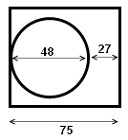Question 7
A company’s profits increased by 12% from 2010 to 2011 and by 18% from 2011 to 2012. By what percent did the company’s profits increase from 2010 to 2012?

 A 10% B 12% C 24% D 30% E 32%
Question 7 Explanation:
The correct answer is (E). The most straightforward method for solving problems of this type is to begin by supplying our own initial value prior to any increase or decrease in profits. Because percents are essentially a division by 100, choosing 100 as our starting value for any percent problem is wise. We are told that for the first year, the percent rose by 12%, so:
100 * 0.12 = 12

So at the start of 2011, the company now has 112, for which there is another increase of 18%, so:
112 * 0.18 = 20.16
Final amount in 2012 = 112 + 20.16 = 132.16

To find the overall percent increase, we find the total increase, then divide this value by the original amount, so:
132.16 − 100 = 32.16
32.16 ÷ 100 = 0.32
= 32%.
 Question 8
What is the product of the matrices: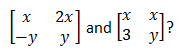AB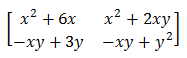C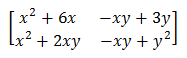DE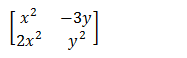Question 8 Explanation:
The correct answer is (B). When asked to find the product of 2 matrices, we must first check to make sure we are capable of doing so. Matrices can be multiplied when the number of columns of the first matrix is equal to the number of rows of the second matrix. Here we have a 2x2 and a 2x2 so the resulting product will be a 2x2 matrix. To calculate the product of 2 2x2 matrices let’s first consider the general form: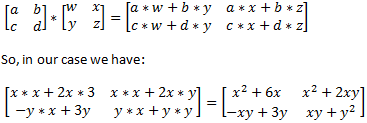Question 9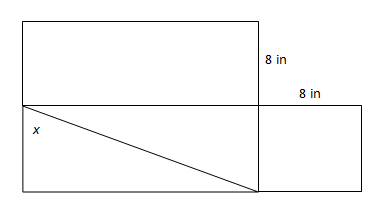The area of the top rectangle is 96 in² and the bottom right figure is a square. In degrees, what is the value of x (rounded to the nearest whole number)?

 A 24 B 33 C 56 D 61 E 68
Question 9 Explanation:
The correct answer is (C). We must start with the provided information to find the relevant pieces of the figure necessary to solve for x in degrees. Using the given area, we can solve for the bottom side length of the right triangle:
96 in² = 8 * Unknown Side Length
Unknown Side Length = 12

We also know, since we are told the shape is a square, that the height of the right triangle is 8 in. We can now solve for the hypotenuse of the triangle using the Pythagorean Theorem:
8² + 12² = hyp²
208 = hyp²
hyp = 14.4

Now, we can set up a relationship between x and the known side lengths:Recall that to solve for x, we must take the sin inverse of both sides: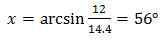Question 10
The function ƒ(x) = cos²x * csc x, can be rewritten as which of the following?

 A sin x * cos x B cos x * cot x C cot x * tan x D sin x * cot x E sec x * csc x
Question 10 Explanation:
The correct answer is (B). Recall that csc x is the same as 1/sin x so we can rewrite this expression as cos x * cos x/sin x and cos x/sin x is the same as cot x so we can rewrite the expression as answer (B), cos x * cot x.
Once you are finished, click the button below. Any items you have not completed will be marked incorrect.
There are 10 questions to complete.
 ← List →